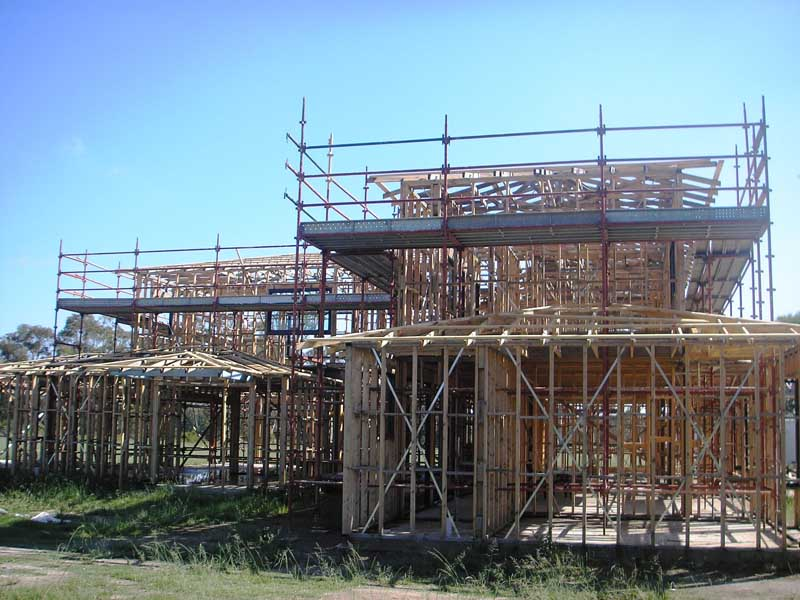## How to calculate the bearing load of the scaffolding?

Time: 2019-04-15
Summary: How to calculate the bearing load of the scaffolding?Where to buy scaffolds means you need to find the right suppliers for your scaffolding products. And knowing some tips on how to work out the bearing load of the scaffolding will do you great favors to find a better one.

The calculation details are listed as follows:
The axle force caused by the standard value of the scaffolding self weight Ng1k : NGK1=0.18kN*14=2.52kN
The axle force caused by the standards value of the scaffolding elements self weight Ng2k : Ng2k=0.176KN*14= 20464KN
The sum of the axle force caused by the construction working load standard valueNqk: For there are working staffs who are walking on two levels of the building at the same time, the standard value of the construction even distribution working load is: qk=2*2kn/m2
Nqk=1.5*(1.0+0.2)*2*2=7.20KN

The even distributed live load calculated above is 7.20KN as the scaffolding belongs to the double line steel tube scaffolding and the load bearing unit separated onto the two standing tubes both external and internal. So the final load bearing value of single tube shall divide 2=3.6KN , Calculation of the designed axle force value of the standing tube for one single tube: N= 1.2+0.85*1.4=1.2*(2.52+2.464)+0.85*1.4*3.6=5.981+4.284=10.265KN